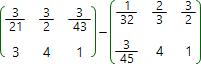# Matrices subtraction online calculatorSubtraction operation can only be applied to the matrices of the same size. The result of the subtraction is the matrix which elements represent the difference of corresponding elements of the matrices being subtracted, i.e. if

C = A B

the elements of matrix C calculated by the formula:

Ci,j = Ai,j Bi,j

Matrix subtraction calculator
A: ×
B: ×A =
B =